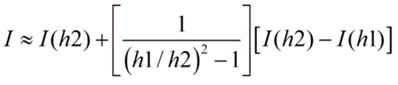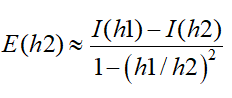Contents - Index

Richardson Extrapolation

Richardson extrapolation is provided as an option when the equation-based Integral function is used with a fixed step size.  A fixed step size can either be specified in one of two ways. The step size can be specified (as the fifth parameter) in the equation-based integral function.  Alternatively, if a step size is not provided in the function, EES will use the step size setting indicated in the Integration tab of the Preferences dialog.  Richardson extrapolation can be employed if the 'Use fixed step size' radio button is selected.

Richardson extrapolation is a clever technique to reduce the truncation error associated with numerical integration.  For a fixed step size, h1, the true value of an integral, I, is approximately equal to

I = I(h1) + E(h1)

where

I(h1) is the value of the integral calculated by using numerical integration with a fixed step size h1

E(h1) is the truncation error resulting from a step size equal to h1

Suppose the integral were calculated again, but with a different step size h2.  In this case,

I = I(h2) + E(h2)

The truncation error for the second-order integration algorithm used in EES is approximately a function of the square of the integration step size soSolving for E(h2) and substituting this result for the integral estimate yieldsThus, the combined result of two numerical integration estimates with differing step sizes can be used to produce an improved estimate.  A more detailed explanation of this technique can be found in Numerical Methods for Engineers by S.C. Chapra and R.P. Canale, McGraw-Hill, 1985.

In the EES implementation of this technique, h2 = h1 / 2 and the extrapolation procedure is applied for every step.  Tests with this extrapolation procedure have shown that it reduces the truncation error by one to two orders of magnitude compared to the error that would occur at the same step size.